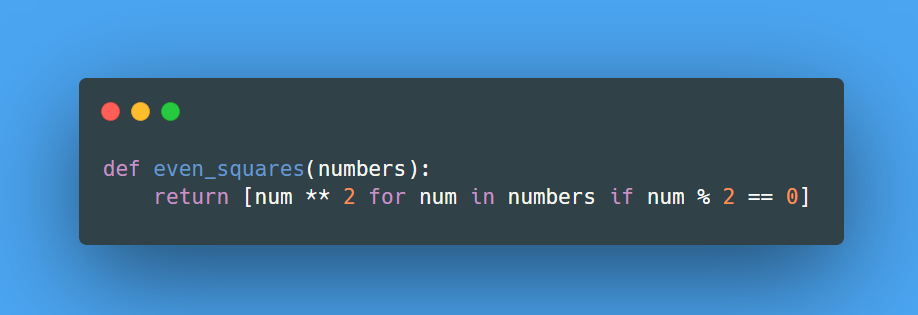Lesson 1 of 0
In Progress

# Even Squares

##### Kedeisha June 15, 2023

Using list comprehension, write a function named `even_squares` that takes a list of integers as input and returns a new list containing the squares of only the even numbers from the input list.

#### Input Data:

• Your function should accept one parameter:
1. `int_list`: [1, 2, 3, 4, 5, 6]

#### Example:

1. If the input is `int_list = [1, 2, 3, 4]`, then the output should be `[4, 16]`.
2. If the input is `int_list = [5, 9, 11]`, then the output should be `[]`.Explanation: The function `even_squares` uses list comprehension to iterate over each element in the input list `numbers` and squares only the even numbers by filtering them using the condition `num % 2 == 0`. The resulting list of squares is then returned.